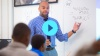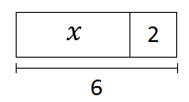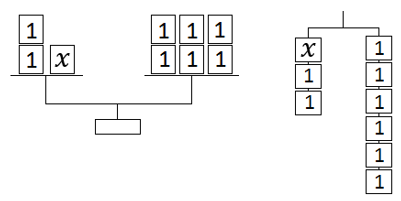# Equations and Inequalities

Students discover how to use equations and inequalities to model relationships between quantities, and investigate the meaning of having a solution to an equation or an inequality.

Math

Unit 6

## Unit Summary

In Unit 6, 6th grade students move from expressions to equations and inequalities. They revisit familiar diagrams such as tape diagrams to model equations, and they discover new models such as balances and hanging mobiles. Students investigate what it means to be a solution to an equation or an inequality and how to use equations and inequalities to model relationships between quantities. When using an equation or inequality to represent real-world situations, students must decontextualize the situation to represent it using variables and symbols and then recontextualize in order to interpret what their answer means in regard to the situation at hand (MP.2). In this unit, students bring concepts from three domains together: Ratios and Proportions, Number Sense, and Expressions and Equations. They re-visit percentages from Unit 2 and solve percent problems using equations. They study relationships between different quantities and draw on their ratio reasoning where relevant. A note on fluency: solving equations provides a good opportunity for students to continue development of and to demonstrate fluency with decimal operations and fraction division. Several problems involve computing with decimals and dividing by fractions; include additional problems in practice for students as needed.

Several prior skills support students in this unit. In 5th Grade Math, students analyzed patterns and relationships when they studied standard 5.OA.3. They also observed what happened when these relationships were plotted on the coordinate plane. In previous 6th-grade units, students studied algebraic and numerical expressions and collections of equivalent ratios. Students draw on all of these concepts and skills in this unit.

There are many future connections to the standards in this unit. In 7th Grade Math, students will deeply investigate proportional relationships in the form $$y=rx$$, understanding the value of $$r$$ as the constant of proportionality. They’ll further investigate the graphs of these equations, and in 8th grade, students will compare across multiple representations of proportional relationships. Students will also become exposed to increasingly more complex equations and inequalities to solve.

Pacing: 17 instructional days (14 lessons, 2 flex days, 1 assessment day)

Fishtank Plus for Math

Unlock features to optimize your prep time, plan engaging lessons, and monitor student progress.## Assessment

The following assessments accompany Unit 6.

### Pre-Unit

Have students complete the Pre-Unit Assessment and Pre-Unit Student Self-Assessment before starting the unit. Use the Pre-Unit Assessment Analysis Guide to identify gaps in foundational understanding and map out a plan for learning acceleration throughout the unit.

### Mid-Unit

Have students complete the Mid-Unit Assessment after lesson 6.

### Post-Unit

Use the resources below to assess student understanding of the unit content and action plan for future units.

Expanded Assessment Package

Use student data to drive your planning with an expanded suite of unit assessments to help gauge students’ facility with foundational skills and concepts, as well as their progress with unit content.

## Unit Prep

### Intellectual Prep

Unit Launch

Prepare to teach this unit by immersing yourself in the standards, big ideas, and connections to prior and future content. Unit Launches include a series of short videos, targeted readings, and opportunities for action planning.#### Internalization of Standards via the Post-Unit Assessment

• Take the Post-Unit Assessment. Annotate for:
• Standards that each question aligns to
• Strategies and representations used in daily lessons
• Relationship to Essential Understandings of unit
• Lesson(s) that Assessment points to

#### Internalization of Trajectory of Unit

• Read and annotate the Unit Summary.
• Notice the progression of concepts through the unit using the Lesson Map.
• Essential Understandings
• Connection to Post-Unit Assessment questions
• Identify key opportunities to engage students in academic discourse. Read through our Teacher Tool on Academic Discourse and refer back to it throughout the unit.

#### Unit-Specific Intellectual Prep

 Model Example Tape diagramBalance/mobile### Essential Understandings

• A solution to an equation or inequality represents the value(s) for the variable that, when substituted in, make the equation or inequality a true statement. The solution set to an inequality can be represented using a ray on a number line.
• Equations are statements of balance between two expressions. In order to solve for a variable in an equation, whatever actions are taken on one side of the equation must also be taken on the other side in order to maintain the balance.
• An equation can be used to model the association between two quantities where one quantity is considered the independent variable and the other quantity is the dependent variable. These relationships can be represented in a table of values and graphed in the coordinate plane.

### Vocabulary

dependent variable

equation

inequality

independent variable

percent equation

solution

substitution

To see all the vocabulary for Unit 6, view our 6th Grade Vocabulary Glossary.

### Materials

• Optional: Balance scale (Teacher set)
• Optional: Graph Paper (2-3 sheets per student)

To see all the materials needed for this course, view our 6th Grade Course Material Overview.

## Lesson Map

Topic A: Reasoning About and Solving Equations

Topic B: Reasoning About and Solving Inequalities

Topic C: Representing and Analyzing Quantitative Relationships

## Common Core Standards

Key

Major Cluster

Supporting Cluster

### Core Standards

#### Expressions and Equations

• 6.EE.B.5 — Understand solving an equation or inequality as a process of answering a question: which values from a specified set, if any, make the equation or inequality true? Use substitution to determine whether a given number in a specified set makes an equation or inequality true.
• 6.EE.B.6 — Use variables to represent numbers and write expressions when solving a real-world or mathematical problem; understand that a variable can represent an unknown number, or, depending on the purpose at hand, any number in a specified set.
• 6.EE.B.7 — Solve real-world and mathematical problems by writing and solving equations of the form x + p = q and px = q for cases in which p, q and x are all nonnegative rational numbers.
• 6.EE.B.8 — Write an inequality of the form x > c or x < c to represent a constraint or condition in a real-world or mathematical problem. Recognize that inequalities of the form x > c or x < c have infinitely many solutions; represent solutions of such inequalities on number line diagrams.
• 6.EE.C.9 — Use variables to represent two quantities in a real-world problem that change in relationship to one another; write an equation to express one quantity, thought of as the dependent variable, in terms of the other quantity, thought of as the independent variable. Analyze the relationship between the dependent and independent variables using graphs and tables, and relate these to the equation. For example, in a problem involving motion at constant speed, list and graph ordered pairs of distances and times, and write the equation d = 65t to represent the relationship between distance and time.

#### Ratios and Proportional Relationships

• 6.RP.A.3 — Use ratio and rate reasoning to solve real-world and mathematical problems, e.g., by reasoning about tables of equivalent ratios, tape diagrams, double number line diagrams, or equations.
• 6.RP.A.3.A — Make tables of equivalent ratios relating quantities with whole number measurements, find missing values in the tables, and plot the pairs of values on the coordinate plane. Use tables to compare ratios.
• 6.RP.A.3.C — Find a percent of a quantity as a rate per 100 (e.g., 30% of a quantity means 30/100 times the quantity); solve problems involving finding the whole, given a part and the percent.

• 6.EE.A.1
• 6.EE.A.2
• 6.EE.A.3

• 5.NF.A.1
• 5.NF.B.3
• 5.NF.B.4

• 5.OA.B.3

• 6.RP.A.1
• 6.RP.A.2
• 6.RP.A.3

• 6.NS.A.1
• 6.NS.B.2
• 6.NS.B.3
• 6.NS.C.6.C
• 6.NS.C.7

• 7.EE.B.4
• 8.EE.B.5
• 8.EE.B.6
• 8.EE.C.7

• 8.F.A.1

• 7.RP.A.2
• 7.RP.A.3

### Standards for Mathematical Practice

• CCSS.MATH.PRACTICE.MP1 — Make sense of problems and persevere in solving them.

• CCSS.MATH.PRACTICE.MP2 — Reason abstractly and quantitatively.

• CCSS.MATH.PRACTICE.MP3 — Construct viable arguments and critique the reasoning of others.

• CCSS.MATH.PRACTICE.MP4 — Model with mathematics.

• CCSS.MATH.PRACTICE.MP5 — Use appropriate tools strategically.

• CCSS.MATH.PRACTICE.MP6 — Attend to precision.

• CCSS.MATH.PRACTICE.MP7 — Look for and make use of structure.

• CCSS.MATH.PRACTICE.MP8 — Look for and express regularity in repeated reasoning.

Unit 5

Numerical and Algebraic Expressions

Unit 7

Geometry

## Request a Demo

See all of the features of Fishtank in action and begin the conversation about adoption.

Yes

No

### Any other information you would like to provide about your school?